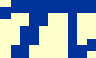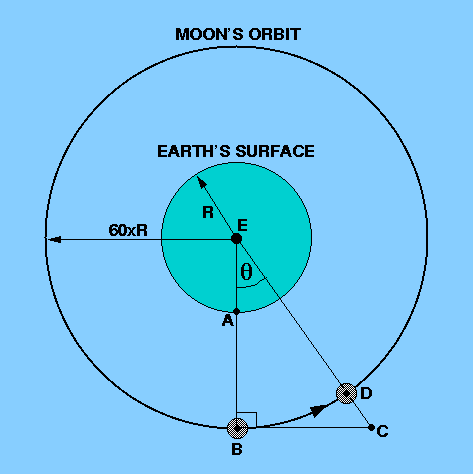Most people know the story of how Newton, at the age of 23, came to think about the motion of the Moon in a way that nobody had ever done before. With extraordinary insight, he constructed an intellectual bridge between the motion of the Moon and the behaviour of falling objects, such as apples from trees.

Newton began to think of the Earth's gravity as extending out to the Moon's orbit. Using his second law of motion, and the fact that the centripetal acceleration, a, of a body moving at speed v in a circle of radius r is given by v2/r, he inferred that the force on a mass m in a circular orbit must be given by

F = ma = mv2/r.

If P is the orbital period of the body, then its speed is

v = 2r / P

and Kepler's third law relates the period and the orbital radius by

P2 = kr3,

where k is the constant of proportionality. Substituting these last two equations into his second law, Newton found

F = 42 m / kr2,

that is, the force on the body is proportional to its mass and inversely proportional to the square of its distance from the central body. According to Newton's third law, the body at the centre of the orbit feels an equal but opposite force, which must be proportional to the mass of the central body (as well as being proportional to the orbiting mass and inversely proportional to the square of its distance). Redefining the constant of proportionality in the above equation (which effectively included the mass of the central body), Newton hence obtained his universal law of gravitation

F = Gm1m2 / r2,

where F is the force of attraction, m1 and m2 are the masses separated by a distance r and G is the constant of proportionality. G is referred to as the (universal) constant of gravitation and has a value of 6.67 x 10-11 Nm2kg-2. Newton's law of gravitation can be stated as follows

Every particle of matter in the universe attracts every other particle of matter with a force directly proportional to the product of the masses and inversely proportional to the square of the distance between them.

In other words, if the mass of the central body doubles, the force between them doubles. If the mass of the central body and the orbiting mass both double, the force between them quadruples. If the distance between the two masses doubles, the force between them is quartered.

It is important to note that this law deals with point masses (i.e. particles). It can be extended to deal with finite bodies by integration, or by application of Gauss's law, from which it is possible to conclude that the gravitational field established outside itself by a spherically symmetric body is the same as it would be if the mass of the body were concentrated at its centre.

 figure 34: Geometry of a small portion of the Moon's orbit around the Earth.In order to test his law of gravitation, Newton applied it to the motion of the Moon. He imagined that if gravity did not exist, the Moon would move through space in a straight line BC, tangent to the orbit at B in Figure 34. But because of the mutual attraction of the Earth and the Moon, the Moon can be thought of as continually falling towards the Earth. Its path, BD, can therefore be thought of as being made up of the straight line motion BC and a fall CD. If the time interval between B and D is one second, then CD is the distance fallen by the Moon in one second.

Now, CD = CE - DE = CE - BE, if we assume the Moon's orbit to be circular. In the right-angled triangle CBE,

BE / CE = cos CEB = cos,    say,

or

CE = BE / cos.

Hence

CD = BE [ (1/cos) - 1 ].

Nowis a very small angle - it is the angle swept out by the Moon's radius vector in one second - so we may use the small angle approximation to write

cos= 1 - (2/2),

whereis in radians. According to the binomial theorem, (1+x)-1=1-x+x2 -x3....., so the equation above can be rewritten as follows:

1 / cos= 1 + (2/2).

Substituting this into the expression for CD, we obtain

CD = BE x2 / 2.

Now, taking the sidereal period of the moon to be 27.322 mean solar days and the number of seconds in a day to be 86400, we have (in radians)= 2/ (27.322 x 86400).

The radius BE of the Moon's orbit is about 240 000 miles = 3.8 x 108 m. Hence it is found that

CD = 1.3 x 10-3 m.

In other words, in 1 second the Moon falls vertically towards the Earth by 1.3 mm. On the other hand, an object which is projected horizontally near the Earth's surface would fall a distance ½gt2 = 4.9 m. The ratio of these two vertical distances is approximately 1/3770. Newton knew that the radius of the Moon's orbit was about 60 times the radius of the Earth. And given that he had just postulated an inverse square law for gravitation, he would have predicted a ratio of 1/3600. In other words, he knew he was right!

In his Principia, Newton went on to explain the polar flattening of the Earth, the precession of the equinoxes due to the Sun and Moon's affect on the Earth's equatorial bulge and the phenomenon of the tides, to name but a few of his great achievements. Newton also combined his laws of motion and gravitation to derive all three of Kepler's empirical laws of planetary motion, placing them on a sound theoretical basis, as we shall now see.